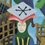# An Algebraist's View: Generalizing Vieta's Formula

It turns out that Vieta's formula applies to any algebraically closed field. Call a field $F$ algebraically closed if(f) every polynomial in $F[x]$ splits over $F$.

Theorem: $\mathbb{C}$ is algebraically closed.

Proof: It suffices to show that there is not a proper finite field extension of $\mathbb{C}$. This is a healthy exercise in Galois theory, and is thus left to the reader. (Hint: Suppose, by way of contradiction, that $L:\mathbb{C}$ is a proper finite extension of $\mathbb{C}$. What can we say about $[L:\mathbb{C}]$?) $\boxed{ }$

Thus, we see that Vieta's formula works in at least one algebraically closed field. Let's broaden the scope.

Theorem: Suppose

$\displaystyle p(x)=\sum_{0\leq k\leq n}\lambda_kx^k \in F[x]$,

where $F$ is an algebraically closed field, and that $p(x)$ splits as

$\displaystyle p(x)=\lambda_n\prod_{1\leq i\leq n}(x-\alpha_i)$.

Then, $\displaystyle \sum_{1\leq i_1.

Proof: A field is necessarily commutative and distributes over addition. Thus, the proof is simply a matter of noting the coefficients must match up. $\boxed{ }$

Thus, we can use Vieta's formula for arbitrary polynomials over fields.

[Edit: Sorry for the awful formatting. I'm used to writing things in pure LaTeX, so this is a little weird to me.]Note by Jacob Erickson
6 years, 9 months ago

This discussion board is a place to discuss our Daily Challenges and the math and science related to those challenges. Explanations are more than just a solution — they should explain the steps and thinking strategies that you used to obtain the solution. Comments should further the discussion of math and science.

When posting on Brilliant:

• Use the emojis to react to an explanation, whether you're congratulating a job well done , or just really confused .
• Ask specific questions about the challenge or the steps in somebody's explanation. Well-posed questions can add a lot to the discussion, but posting "I don't understand!" doesn't help anyone.
• Try to contribute something new to the discussion, whether it is an extension, generalization or other idea related to the challenge.

MarkdownAppears as
*italics* or _italics_ italics
**bold** or __bold__ bold
- bulleted- list
• bulleted
• list
1. numbered2. list
1. numbered
2. list
Note: you must add a full line of space before and after lists for them to show up correctly
paragraph 1paragraph 2

paragraph 1

paragraph 2

[example link](https://brilliant.org)example link
> This is a quote
This is a quote
    # I indented these lines
# 4 spaces, and now they show
# up as a code block.

print "hello world"
# I indented these lines
# 4 spaces, and now they show
# up as a code block.

print "hello world"
MathAppears as
Remember to wrap math in $$ ... $$ or $ ... $ to ensure proper formatting.
2 \times 3 $2 \times 3$
2^{34} $2^{34}$
a_{i-1} $a_{i-1}$
\frac{2}{3} $\frac{2}{3}$
\sqrt{2} $\sqrt{2}$
\sum_{i=1}^3 $\sum_{i=1}^3$
\sin \theta $\sin \theta$
\boxed{123} $\boxed{123}$

Sort by:

Nice bro! :D

- 6 years, 5 months ago

@Jacob Erickson Can you add this to a suitable skill in the Vieta Formula Wiki? Let me know if you think a different skill would be suitable.

Staff - 5 years, 11 months ago